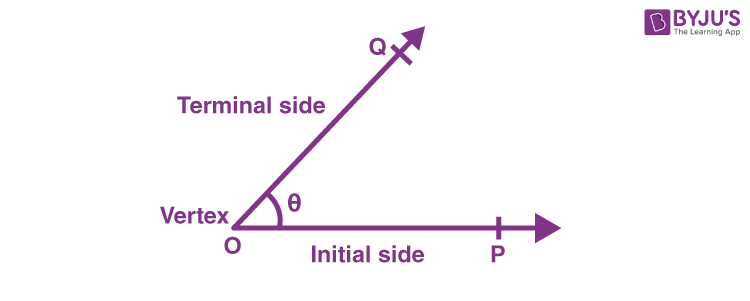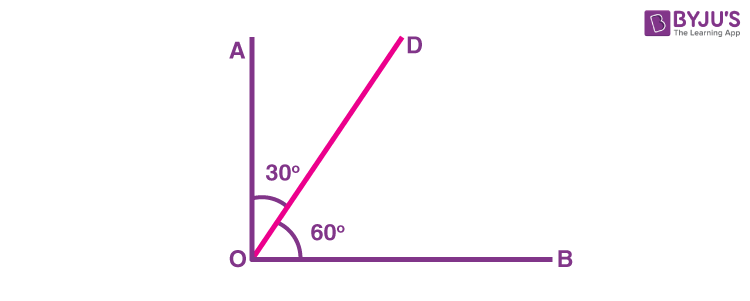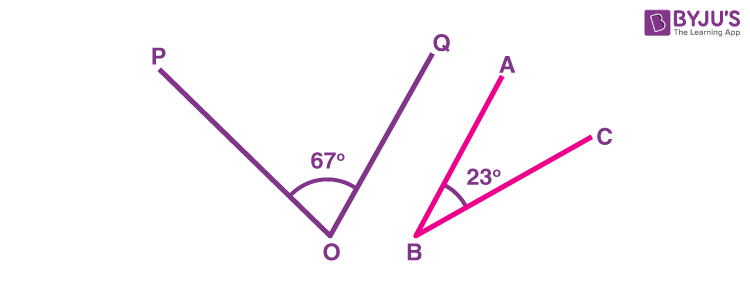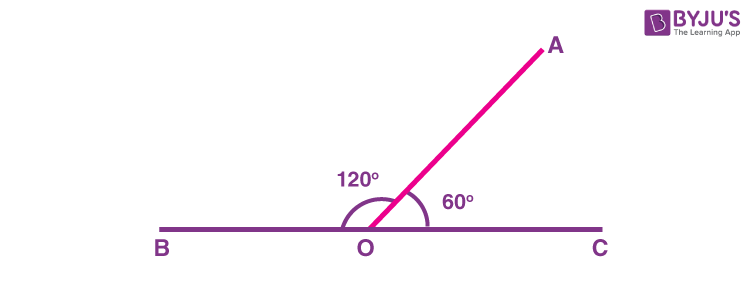# Supplementary And Complementary Angles

Supplementary angles and complementary angles are defined with respect to the addition of two angles. If the sum of two angles is 180 degrees then they are said to be supplementary angles, which form a linear angle together. Whereas if the sum of two angles is 90 degrees, then they are said to be complementary angles, and they form a right angle together.

When two line segments or lines meet at a common point (called a vertex), at the point of intersection an angle is formed. When a ray is rotated about its endpoint, then the measure of its rotation in an anti-clockwise direction is the angle formed between its initial and final position.In fig. 1 if the ray OP is rotated in the direction of the ray OQ, then the measure of its rotation represents the angle formed by it. In this case, the measure of rotation which is the angle formed between the initial side and the terminal side is represented by Ɵ.

## Complementary Angles

When the sum of two angles is 90°, then the angles are known as complementary angles. In other words, if two angles add up to form a right angle, then these angles are referred to as complementary angles. Here we say that the two angles complement each other.

### How to Find Complementary Angles?

Suppose if one angle is x then the other angle will be 90° – x. Hence, we use these complementary angles for trigonometry ratios, where one ratio is complementary to another ratio by 90 degrees such as;

• sin (90° – A) = cos A and cos (90° – A) = sin A
• tan (90° – A) = cot A and cot (90° – A) = tan A
• sec (90° – A) = cosec A and cosec (90° – A) = sec A

Hence, you can see here the trigonometric ratio of the angles gets changed if they complement each other.In the above figure, the measure of angle BOD is 60° and angle AOD measures 30°. By adding both of these angles we get a right angle, therefore ∠BOD and ∠AOD are complementary angles.

The following angles in Fig. 3 given below are complementary to each other as the measure of the sum of both the angles is 90°. ∠POQ and ∠ABC are complementary and are called complements of each other.For example: To find the complement of 2x + 52°, subtract the given angle from 90 degrees.

90o –  (2x + 52o) =  90o – 2x – 52o  = -2x + 38o

The complement of 2x + 52o is 38o – 2x.

 Facts of complementary angles: Two right angles cannot complement each other Two obtuse angles cannot complement each other Two complementary angles are acute but vice versa is not possible

## Supplementary Angles

When the sum of two angles is 180°, then the angles are known as supplementary angles. In other words, if two angles add up, to form a straight angle, then those angles are referred to as supplementary angles.

### How to Find Supplementary Angles?

The two angles form a linear angle, such that, if one angle is x, then the other the angle is 180° – x. The linearity here proves that the properties of the angles remain the same. Take the examples of trigonometric ratios such as;

• Sin (180 – A) = Sin A
• Cos (180 – A) = – Cos A (quadrant is changed)
• Tan (180 – A) = – Tan AIn Fig. 4 given above, the measure of ∠AOC is 60o and ∠AOB measures 120o. By adding both of these angles we get a straight angle. Therefore, ∠AOC and ∠AOB are supplementary angles, and both of these angles are known as a supplement to each other.

Also, learn:

### Difference between Complementary and Supplementary Angles

 Complementary Angles Supplementary Angles Sum is equal to 90 degrees Sum is equal to 180 degrees Two angles complement each other Two angles supplement each other These angles do not form linear pair of angles These angles form linear pair of angles Meant only for right angles Meant only for straight angles

How to remember easily the difference between Complementary angles and supplementary angles?

• C letter of Complementary stands for “Corner” (A right angle, 90°)
• S letter of Supplementary stands for “Straight” ( a straight line, 180°)

## Video Lesson on Types of Angles## Solved Examples

The example problems on supplementary and complementary angles are given below:

Example 1:

Find the complement of 40 degrees.

Solution:

As the given angle is 40 degrees, then,

The complement is 50 degrees.

We know that sum of complementary angles =  90 degrees

So, 40° + 50° = 90°

Example 2:

Find the supplement of the angle 1/3 of 210°.

Solution:

Step 1: Convert 1/3 of 210°

That is, (1/3) x 210° = 70°

Step 2: Supplement of 70° = 180° – 70° = 110°

Therefore, the supplement of the angle 1/3 of 210° is 110°

Example 3:

The measures of the two angles are (x + 25)° and (3x + 15)°. Find the value of x if angles are supplementary angles.

Solution:

We know that, Sum of Supplementary angles =  180 degrees

So,

(x + 25)° + (3x + 15)° = 180°

4x + 40°  = 180°

4x = 140°

x = 35°

The value of x is 35 degrees.

Example 4:

The difference between the two complementary angles is 52°. Find both the angles.

Solution:

Let, First angle = m degrees, then,

Second angle =  (90 – m)degrees   {as per the definition of complementary angles}

Difference between angles = 52°

Now,

(90° – m) – m = 52°

90° – 2m = 52°

– 2m = 52° – 90°

-2m = -38°

m = 38°/2°

m = 19°

Again, second angle = 90° – 19°  = 71°

Therefore, the required angles are 19°, 71°.

### Practice Questions

1. Check if 65° and 35° are complementary angles.
2. Are 80° and 100° supplementary?
3. Find the complement of 54°.
4. Find the supplement of 99°.
5. If one angle measures 50° and is supplementary to another angle. Then find the value of another angle. Also, state what type of angle it is?

## Frequently Asked Questions – FAQs

Q1

### What are complementary angles? Give example.

When the sum of two angles is equal to 90 degrees, they are called complementary angles. For example, 30 degrees and 60 degrees are complementary angles.
Q2

### What are supplementary angles? Give examples.

When the sum of the measure of two angles is equal to 180 degrees, they are called supplementary angles. For example, 70 degrees and 110 degrees are supplementary.
Q3

### How to find complementary angles?

Since the sum of complementary angles equals 90 degrees, therefore if we know the measure of one angle, then we can find the unknown angle easily.
For example, if one of the two angles is 45 degrees, then;
x + 45 = 90
x = 90 – 45 = 45°
Q4

### What is the complementary angle of 40 degrees?

The complementary angle of 40 degrees is:
90 – 40 = 50 degrees
Q5

### How to find supplementary angles?

To find the angle which is supplementary to another angle, subtract the given angle from 180 degrees.
For example, if one angle is 60 degrees, then another angle is 180 – 60 = 120 degrees.
Quiz on Supplementary Complementary Angles

1. My question is in Maths Class 7 : “What is the supplement of 2x-13?”

• Supplementary angles sum up to 180 degrees.
Therefore,
2x-13 = 180
2x = 180+13
x = 193/2
x = 96.5 degrees

• What is the complementary angle of 60° ?

• The complementary angle of 60° is 30°
60°+30° = 90°

2. What is the complement of 40°

• The complement of 40° is 50°
Since 90° – 40° = 50°

3. Let x and y are the two angles, supplementary to each other.
As per the statement, say, y = 2x
Therefore,
x+y = 180 degrees (supplementary angles)
x+2x = 180
3x = 180
x = 180/3 = 60
Hence, x = 60 degrees, y = 2x = 2(60) = 120 degrees